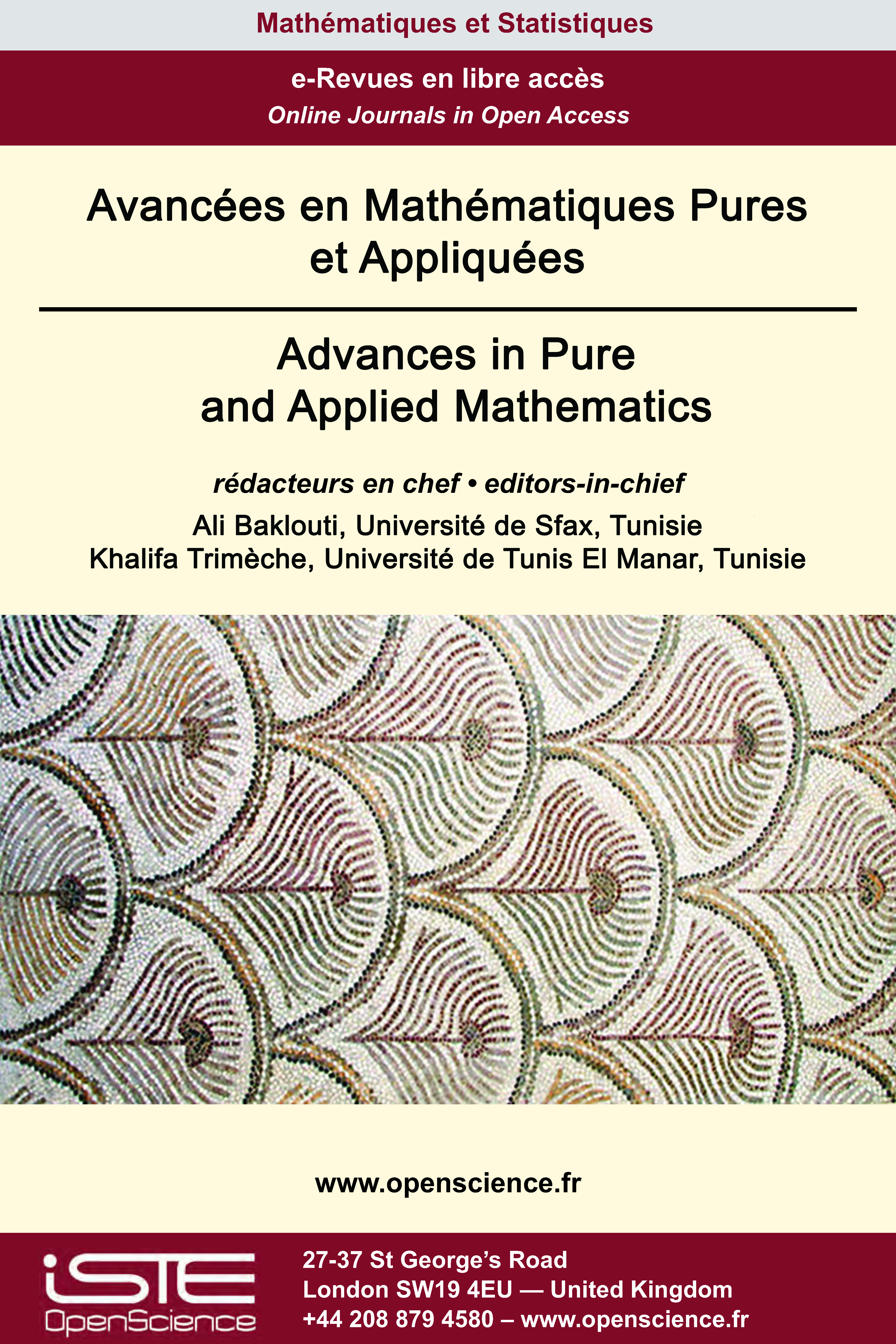# Vol 12 - Numéro 1 (Janvier 2021)

## Articles parus

Régions invariantes et existence globale de solutions pour un système de réaction-diffusion généralisé à m-composants avec une matrice de diffusion tridiagonale de Toeplitz symétrique

The aim of this paper is to construct invariant regions of a generalized m-component reaction-diffusion system with tridiagonal symmetric Toeplitz diffusion matrix and nonhomogeneous boundary conditions and polynomial growth for the nonlinear reaction terms. Using the eigenvalues and eigenvectors of the diffusion matrix and the parabolicity conditions. So we prove the global existence of solutions using Lyapunov functional.

Problème de la valeur initiale pour le système dynamique des gaz à pression nulle non conservateur

In this article, we study initial value problem for the zero-pressure gas dynamics system in non conservative form and the associated adhesion approximation. We use adhesion approximation and modi-ed adhesion approximation in the construction of weak asymptotic solution. First we prove a general existence result for the adhesion model for the initial velocity component in $H^s \mbox{ for } s$ > $\frac{n}{2} + 1$ and the initial data for the density component being a $C^1$ function. Using this, we construct weak asymptotic solution for the system with initial velocity in $L^2 \cap L^{\infty}$ and the initial density being a bounded Borel measure. Then we make a detailed analysis of the explicit formula for the weak asymptotic solution and generalized solution for the plane-wave type initial data.

Sur l’existence de solutions d’un problème biharmonique non local

This paper is concerned with the existence of an eigenvalue for a p(x)-biharmonic Kirchhoff problem with Navier boundary condition. Under some suitable conditions, we establish that any λ > 0 is an eigenvalue . The proofs combine variational methods with energy estimates. The main results of this paper improve and generalize the previous one introduced by Kefi and Rădulescu (Atti Accad. Naz. Lincei Cl. Sci. Fis. Mat. Natur. 29 (2018), 439-463).

Marche aléatoire sur les extensions finies de réseaux

We obtain a precise formula for the probability that a random walker returns to the origin after n steps on some semi-direct product groups obtained by extending a Euclidean lattice by a finite group. Prior to this work, to the best of our knowledge, for the class of groups considered, only asymptotic estimates were available in the literature.### Autres numéros :

2020

Volume 20- 11

Numéro 1 (Mai 2020)
Numéro 2 (Septembre 2020)

À paraître

2021

Volume 21- 12

Numéro 1 (Janvier 2021)
Numéro 2 (Mai 2021)